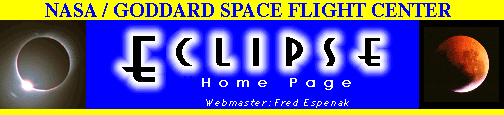# Total Solar Eclipse of 1998 February 26

## Table 1 - Elements of the Eclipse```
Geocentric Conjunction      17:36:39.82 TDT     J.D. = 2450871.233794
of Sun & Moon in R.A.:   (=17:35:36.42 UT)

Instant of            17:29:26.62 TDT     J.D. = 2450871.228780
Greatest Eclipse:      (=17:28:23.22 UT)

Geocentric Coordinates of Sun & Moon at Greatest Eclipse (DE200/LE200):

Sun:       R.A. = 22h38m18.927s       Moon:      R.A. = 22h38m02.542s
Dec. =-08°36'05.03"                   Dec. =-08°22'08.48"
Semi-Diameter =    16'09.09"          Semi-Diameter =    16'35.32"
Eq.Hor.Par. =        8.88"            Eq.Hor.Par. =  1°00'52.61"
Delta R.A. =        9.437s/h         Delta R.A. =      145.611s/h
Delta Dec. =       56.21"/h          Delta Dec. =      641.43"/h

Lunar Radius   k1 = 0.2725076 (Penumbra)        Shift in      delta b =  0.00"
Constants:    k2 = 0.2722810 (Umbra)       Lunar Position:   delta l =  0.00"

Geocentric Libration:   l =  -2.4°       Brown Lun. No. = 1214
(Optical + Physical)    b =  -0.2°         Saros Series =  130 (51/73)
c = -23.5°            Ephemeris = (DE200/LE200)

Eclipse Magnitude = 1.04411        Gamma = 0.23909    Delta T =    63.4 s

Polynomial Besselian Elements for:   1998 Feb 26   17:00:00.0 TDT  (=t0)

n        x          y         d          l1         l2          Á

0  -0.3388351  0.1505067 -8.6094313  0.5391787 -0.0069440  71.779228
1   0.5544966  0.1611319  0.0152151 -0.0000521 -0.0000519  15.003132
2   0.0000103  0.0000494  0.0000017 -0.0000128 -0.0000128   0.000002
3  -0.0000092 -0.0000026  0.0000000  0.0000000  0.0000000   0.000000

Tan f1 = 0.0047215    Tan f2 = 0.0046980

At time "t1" (decimal hours), each Besselian element is evaluated by:

a = a0 + a1*t + a2*t2 + a3*t3    (or a = À [an*tn]; n = 0 to 3)

where:  a = x, y, d, l1, l2, or Á
t = t1 - t0  (decimal hours) and t0 = 17.000 TDT

The Besselian elements were derived from a least-squares fit to elements
calculated at five separate times over a six hour period centered at t0.
Thus the Besselian elements are valid over the period 14.00 < t0 < 20.00 TDT.

Saros Series 130:  Member 51 of 73 eclipses in series.

```

Table adapted from NASA RP 1383 "Total Solar Eclipse of 1998 February 26".WebMaster: Fred Espenak
Planetary Systems Branch - Code 693
e-mail: espenak@lepvax.gsfc.nasa.gov

NASA/Goddard Space Flight Center, Greenbelt, Maryland 20771 USALast revised: 1997 April 23 - F. Espenak